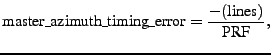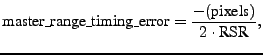Next: M_OVS Up: M_TIMING Previous: Output Description   Contents

# Implementation

The master timing error is computed in three steps:

First for each window position, the (zero meaned) master amplitude window is shifted over the (zero meaned) simulated amplitude and the correlation is obtained (see equation D.24) by computing all pointwise products and dividing by the norms of the particular windows.

Second, we select the most frequently occurring line and pixel offset pair by searching for the highest frequency of window offsets above the average correlation.

Finally, we convert the obtained integer line and pixel offset to the master timing error using the following formulation(G1)(G2)

where PRF is the pulse repetition frequency and RSR is the one-way resampling rate in Hz.

The algorithms which are used to compute correlation are the same as the ones used in the step COARSE_CORR, see the related chapter for details on the magspace and magfft methods.Next: M_OVS Up: M_TIMING Previous: Output Description   Contents
Leijen 2009-04-14